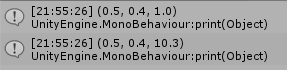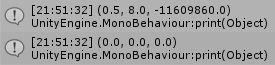# manual MVP projection gives different results then WorldToViewportPoint

I’m trying to manually perform an MVP transformation for a single point. I think (hope) I have it sort of figured out, except for one quirk: my Z coordinate in Viewport space is off.

Here is the code:

``````var pos = Vector3.zero;
var pos_homogeneous_coordinates = new Vector4(pos.x, pos.y, pos.z, 1);
var pos_local = cam.transform.localToWorldMatrix.inverse * pos_homogeneous_coordinates;
pos_local.z *= -1; // unity uses a left-handed coordinate system for the projection
var pos_in_clip_space = cam.projectionMatrix * pos_local;
var pos_in_NDC = (pos_in_clip_space / pos_in_clip_space.w);
var pos_in_viewport_space = (Vector3) ((pos_in_NDC + new Vector4(1,1,1,0)) / 2f);

print(pos_in_viewport_space);
print(cam.WorldToViewportPoint(pos));
``````

where `cam` is the camera. The result of this in my case is:And I would expect them to be identical. Also, my code seems to produce strange results in edge cases or when the position is outside the clipping area. E.g. using `pos = cam.transform.position`:My question simply is where I am going wrong, as I don’t see it. Any pointers or help is highly appreciated =)

The strange behavior is actually not very strange at all. After consulting the documentation of `WorldToViewportPoint` (again) I found

Viewport space is normalized and relative to the camera. The bottom-left of the camera is (0,0); the top-right is (1,1). The z position is in world units from the camera.

After further investigation, I also found that this distance is measured as the normal distance between the point and the projection plane.

In other words, both ways are correct. Unity gives an absolute difference, I return the normalized distance (which may be a lot less meaningful since it usually is `1`.

Regarding the strange behavior around `cam.transform.position` I picked the most unfortunate point to look at. When `z --> 0` (distance between camera and point), `w --> 0`; thus, at the camera’s origin one would divide by 0 which is not a good idea.